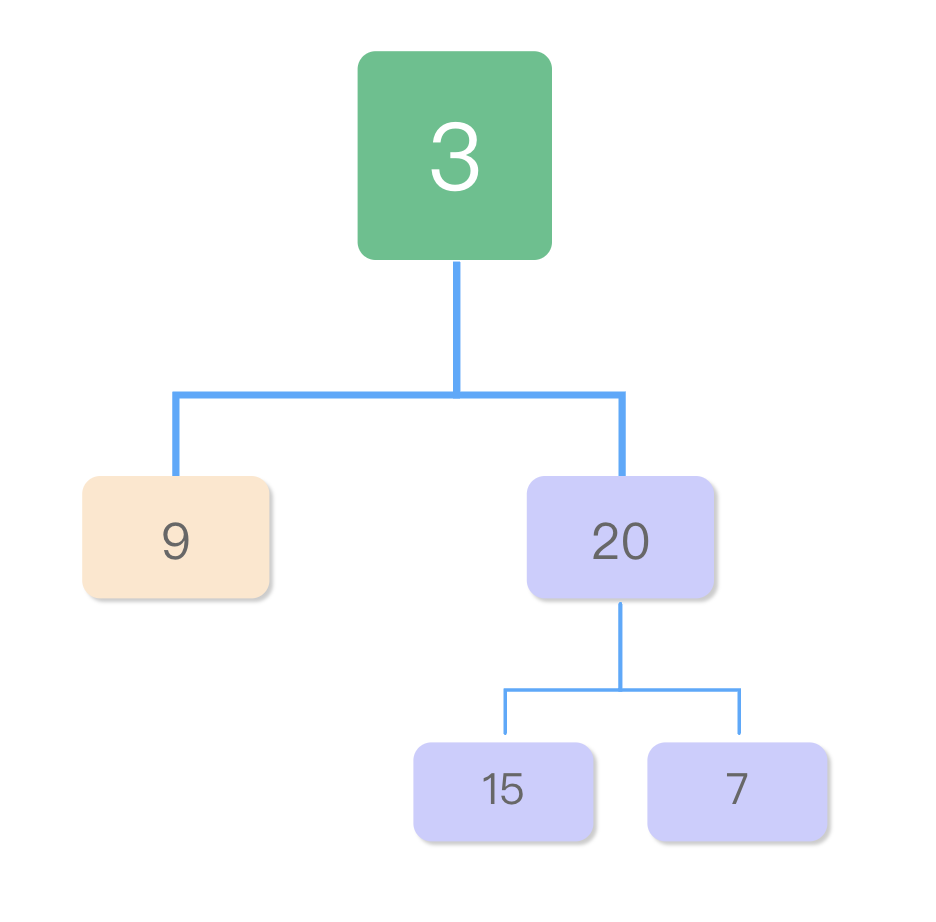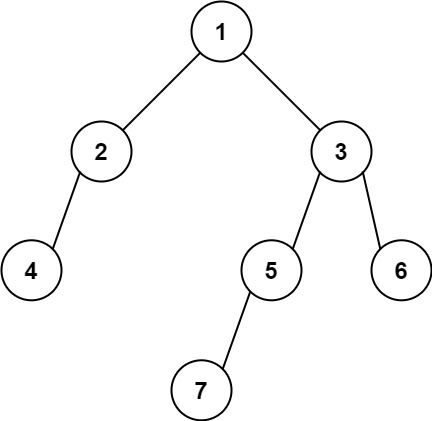# 将leetcode中二叉树的数组结构转为真实的树结构

## 1. javascript 版本

```COPYJAVASCRIPT```function TreeNode(val) {
this.val = val;
this.left = this.right = null;
}``````

```COPYJAVASCRIPT```const array2binary = (arr) => {
if (!arr || !arr.length) {
return null;
}
let index = 0;
const queue = [];
const len = arr.length;

while (index < len) {
index++;
const parent = queue.shift();
if (arr[index] !== null && arr[index] !== undefined) {
const node = new TreeNode(arr[index]);
parent.left = node;
queue.push(node);
}

index++;
if (arr[index] !== null && arr[index] !== undefined) {
const node = new TreeNode(arr[index]);
parent.right = node;
queue.push(node);
}
}
};``````

`COPYJAVASCRIPT`const root = array2binary([3, 9, 20, null, null, 15, 7]);``## 2.C++ 版本

```COPYCPP```struct TreeNode {
int val;
TreeNode *left;
TreeNode *right;

TreeNode() : val(0), left(nullptr), right(nullptr) {}
TreeNode(int x) : val(x), left(nullptr), right(nullptr) {}
TreeNode(int x, TreeNode *left, TreeNode *right) : val(x), left(left), right(right) {}
};
``````

```COPYCPP```/**
* 将leetcode中的数组转为二叉树
* 因C++中的vector只能是同一个类型，这里用INT_MAX来代替null
* @param {vector<int>} nums
* @return {TreeNode}
*/
TreeNode *vectorToTree(vector<int> nums) {
int i = 1;
bool isLeftTree = true; // 是否是左分支，true为左分支，false时是右分支

if (nums.size() == 0) {
return nullptr;
}
queue<TreeNode *> myQueue;

while (i < nums.size()) {
TreeNode *topNode = myQueue.front();

// 这里我们与js版稍微有点区别
// 队列中的节点只要用两次，当isLeftTree指向到右分支时，说明可以取出了
if (!isLeftTree) {
myQueue.pop();
}

// 我们用INT_MAX来标识nullptr
if (nums[i] != INT_MAX) {
TreeNode *node = new TreeNode(nums[i]);

if (isLeftTree) {
topNode->left = node;
} else {
topNode->right = node;
}
myQueue.push(node);
}
isLeftTree = !isLeftTree;
i++;
}
}``````

```COPYCPP```vector<int> nums = {3, 9, 20, INT_MAX, INT_MAX, 15, 7};

TreeeNode *root = vectorToTree(nums);``````

## 4. 题外话

```COPYJAVASCRIPT```// 错误代码，请不要直接使用
const array2binary = (arr, index = 0) => {
if (!arr || arr[index] === null || index >= arr.length) {
return null;
}
const node = new TreeNode(arr[index]);
node.left = array2binary(arr, index * 2 + 1);
node.right = array2binary(arr, index * 2 + 2);

return node;
};``````

```COPYSHELL```输入: [1,2,3,4,null,5,6,null,null,7]### 公众号：## 相关文章

### 公众号：